Related Articles
Class 10 NCERT Solutions- Chapter 2 Polynomials – Exercise 2.3
• Last Updated : 09 Mar, 2021

### Question 1. Divide the polynomial p(x) by the polynomial g(x) and find the quotient and remainder in each of the following:

(i) p(x) = x3 – 3x2 + 5x – 3, g(x) = x2 – 2

(ii) p(x) = x4 – 3x2 + 4x + 5, g(x) = x2 + 1 – x

(iii) p(x) = x4– 5x + 6, g(x) = 2 – x2

Solution:

i) p(x) = x3 – 3x2 + 5x – 3, g(x) = x2 – 2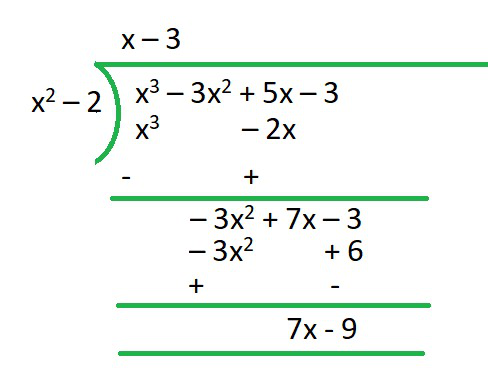R = 7x-9

Q = x-3

ii) p(x) = x4 – 3x2 + 4x + 5, g(x) = x2 + 1 – x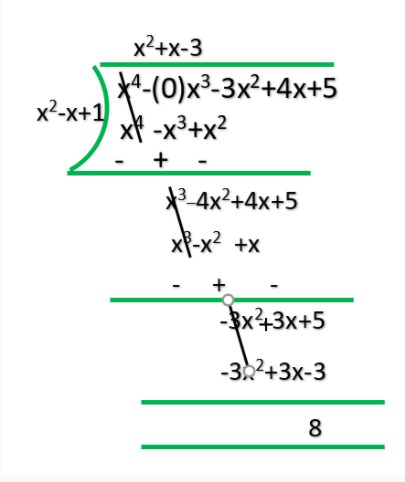R = 8

Q = x2+x-3

iii) p(x) = x4– 5x + 6, g(x) = 2 – x2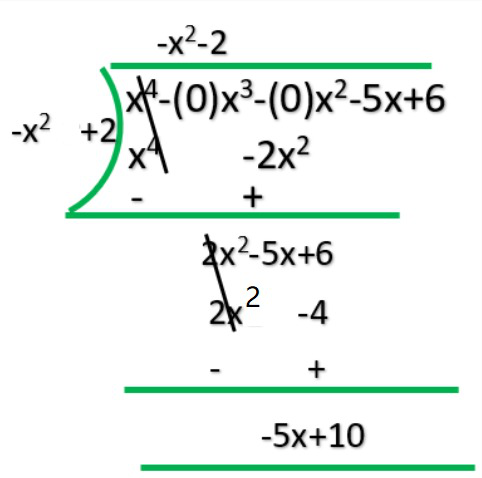Q = -x2-2

R = -5x+10

### Question 2. Check whether the first polynomial is a factor of the second polynomial by dividing the second polynomial by the first polynomial.

(i) t2 – 3, 2t4 + 3t3 – 2t2– 9t – 12

(ii) x2 + 3x + 1, 3x4 + 5x3 – 7x2 + 2x + 2

(iii) x3 – 3x + 1, x5 – 4x3 + x2 + 3x + 1

Solution:

i) t2 – 3, 2t4 + 3t3 – 2t2– 9t – 12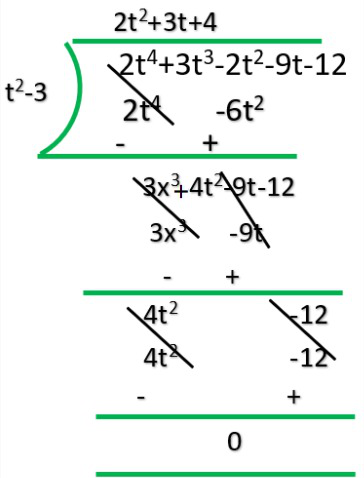Q = 2t3+3t+4

R = 0

Yes 1st polynomial is factor of 2nd polynomial.

ii) x2 + 3x + 1, 3x4 + 5x3 – 7x2 + 2x + 2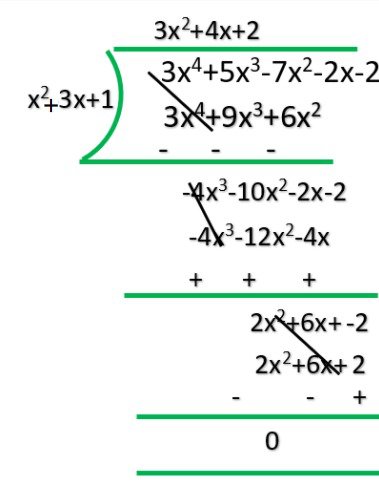R = 0

Q = 3x2-4x+2

iii) x3 – 3x + 1, x5 – 4x3 + x2 + 3x + 1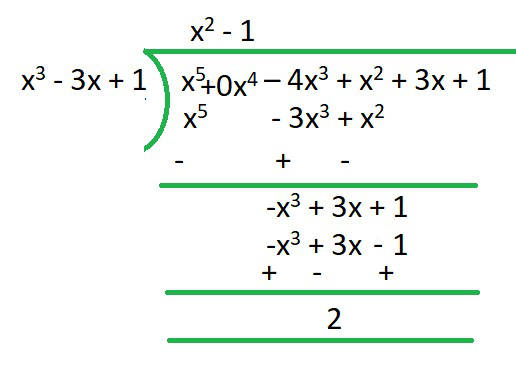R = x2-1

Q = 2

### Question 3. Obtain all other zeroes of 3x4 + 6x3 – 2x2 – 10x – 5, if two of its zeroes are and √(5/3) and -√(5/3).

Solution: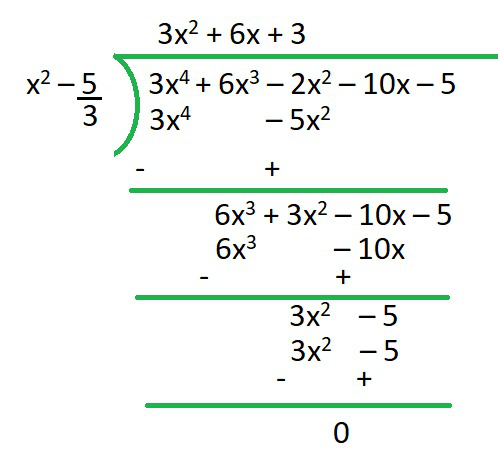R = 0

Q = 3x2+6x+3

∴ we are factorizing

3x2+6x+3

x2+2x+1

(x+1)2

(x+1)  (x+1) = 0

∴ x = -1 and x = -1### Question 4. On dividing x3 – 3x2 + x + 2 by a polynomial g(x), the quotient and remainder were x – 2 and -2x + 4 respectively. Find g(x).

Solution:

Dividend = Divisor * Quotient + Remainder

x3-3x2+3x-2/x-2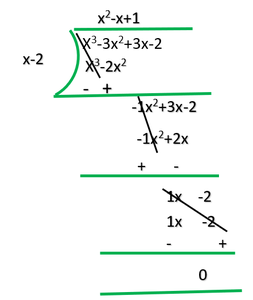R = 0

Q = x2 -x +1

### Question 5. Give examples of polynomials p(x), g(x), q(x) and r(x), which satisfy the division algorithm and:

(i) deg p(x) = deg q(x)

(ii) deg q(x) = deg r(x)

(iii) deg r(x) = 0

Solution:

i) deg p(x) = deg q(x)

p(x)=2x2-2x+14, g(x)=2

p(x)/g(x)=2x2-2x+14/2=(x2-x+7)

=x2-x+7=q(x)

=q(x)=x2-x+7

r(x)=0

ii) deg q(x)=deg r(x)

p(x)=4x2+4x+4, g(x)=x2+x+1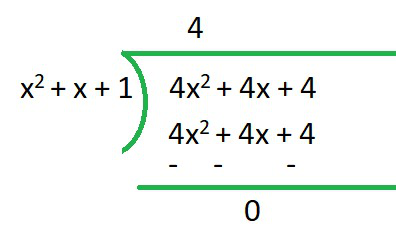q(x) = 4

r(x) = 0

∴Here deg q(x)=deg r(x)

iii) deg r(x)=0

p(x)=x3+2x2-x+2 ,g(x)=x2-1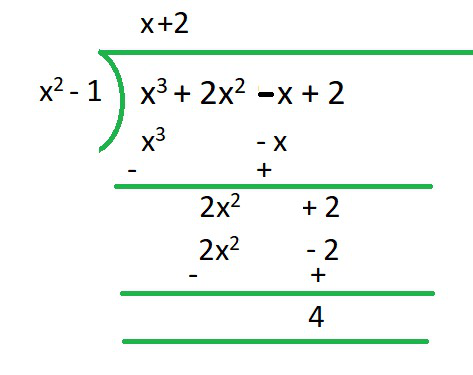q(x) = x+2

r(x) = 4

deg of r(x) = 0

My Personal Notes arrow_drop_up